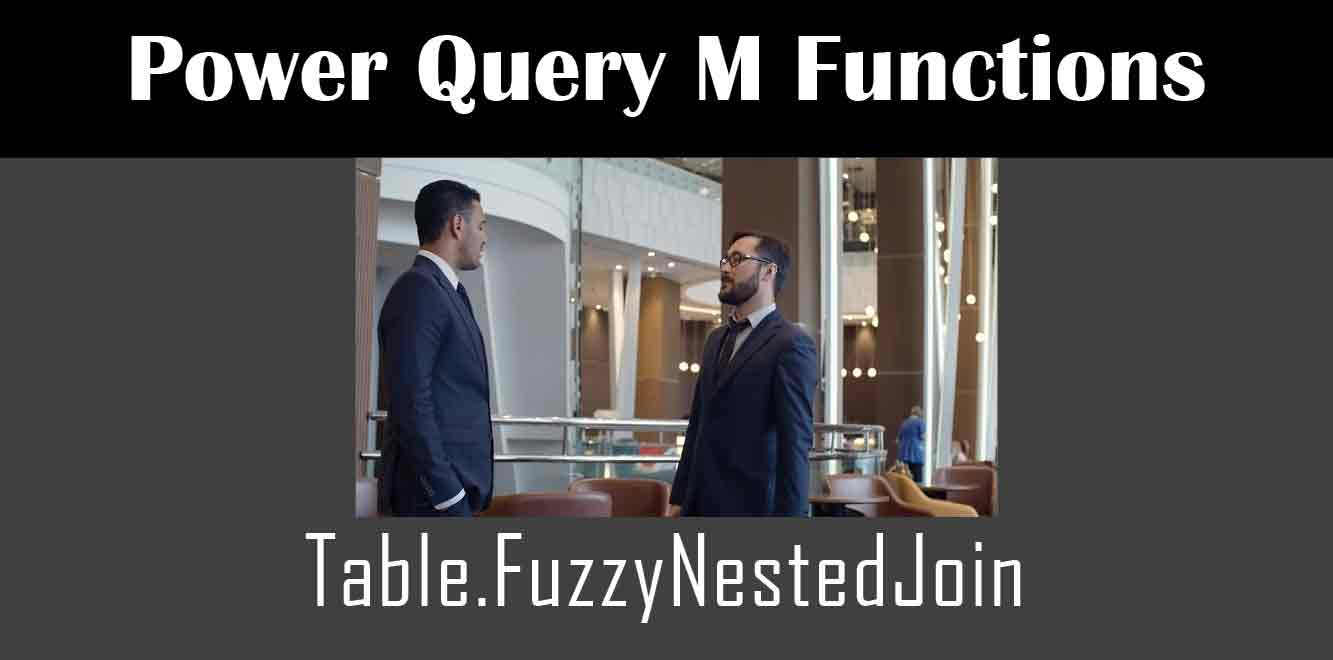# Table.FuzzyNestedJoin

## ### What is the Table.FuzzyNestedJoin Function?

The Table.FuzzyNestedJoin function is a type of join that matches two tables based on a fuzzy match of one or more columns. This function is similar to the regular Nested Join function in Power Query, but it allows for partial matches and can handle differences in casing, spelling, and other minor variations.

The Table.FuzzyNestedJoin function takes four arguments:

1. Table1: The first table to join.

2. JoinColumnName1: The name of the column in Table1 to use for the join.

3. Table2: The second table to join.

4. JoinColumnName2: The name of the column in Table2 to use for the join.

The function then compares the values in the two join columns and returns a new table that contains all the columns from both tables where there is a fuzzy match on the join columns.

## The M Code Behind Table.FuzzyNestedJoin

The M code behind the Table.FuzzyNestedJoin function is complex and can be intimidating for beginners. However, understanding how this code works can help you to customize the function and apply it to your own datasets.

The code for the Table.FuzzyNestedJoin function is as follows:

``` let FuzzyJoin = (t1 as table, joincol1 as text, t2 as table, joincol2 as text) => let CleanAndSplit = (str as text) => Text.Split(Text.Replace(Text.Lower(str),"[^a-z]","")," "), StrToList = (str as text) => List.Distinct(CleanAndSplit(str)), ListToTable = (list as list) => Table.FromList(list, type table [Word = text]), TableToList = (table as table) => List.Sort(Table.Column(table,"Word")), CombineText = (table as table) => Text.Combine(Table.Column(table,"Word")," "), Source1 = Table.AddColumn(t1,"JoinKey",each CleanAndSplit(Text.Trim(Text.Lower(Text.From(_[joincol1]))))), Source2 = Table.AddColumn(t2,"JoinKey",each CleanAndSplit(Text.Trim(Text.Lower(Text.From(_[joincol2]))))), Join = Table.NestedJoin(Source1,"JoinKey",Source2,"JoinKey","Joined",JoinKind.FullOuter), Expand = Table.ExpandTableColumn(Join,"Joined"), Filter = Table.SelectRows(Expand, each ([JoinKey] <> null)), Group = Table.Group(Filter,"JoinKey",{"Grouped", each CombineText(ListToTable([Joined][Word])), type text}), Result = Table.ExpandTableColumn(Group,"Grouped") in Result in FuzzyJoin ```

As you can see, the code is quite long and involves several helper functions. Let’s break down each part of the code to see how it works.

### Cleaning and Splitting Text

The first helper function in the code is CleanAndSplit. This function takes a text string, converts it to lowercase, removes any non-alphabetic characters, and splits it into individual words. This is done to make the text more standardized and easier to compare.

### Converting Text to a List and Table

The next two helper functions, StrToList and ListToTable, convert a list of words into a table with a single column called “Word”. This is done so that we can use the Table.NestedJoin function to match the words in the two tables.

### Combining Text

The CombineText function takes a table of words and combines them back into a single text string. This is done so that we can display the matched values in a single column in the resulting table.

The Source1 and Source2 variables add a new column to each table called “JoinKey”. This column contains a list of cleaned and split words from the join column in each table.

### Performing the Nested Join

The Join variable performs the Nested Join by matching the values in the JoinKey columns of the two tables. This produces a new table with a single column called “Joined” that contains all the rows from both tables where there is a fuzzy match on the join columns.

### Expanding the Nested Join

The Expand variable expands the “Joined” column back into the original columns from both tables. This creates a new table with all the columns from both tables where there is a fuzzy match on the join columns.

### Filtering Null Values

The Filter variable removes any rows from the resulting table where the JoinKey column is null. This ensures that only rows where there is a fuzzy match on the join columns are included in the final table.

### Grouping the Results

The Group variable groups the rows in the resulting table by their JoinKey value. It then combines the words from the “Joined” column for each group into a single text string using the CombineText function.

### Expanding the Grouped Results

The Result variable expands the “Grouped” column back into the original columns from both tables to produce the final table with all the columns from both tables where there is a fuzzy match on the join columns.

The Table.FuzzyNestedJoin function is a powerful tool for matching columns between two tables in Power Query. While the M code behind this function may be complex, understanding how it works can help you to apply it to your own datasets and customize it to meet your specific needs.

Subject